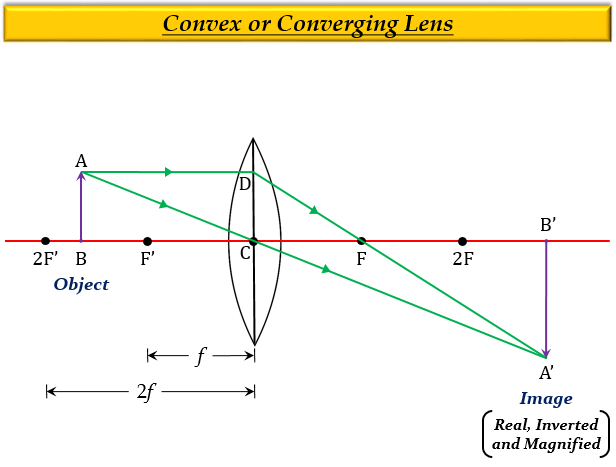# To obtain a magnification of, $-$2 with a convex lens of focal length 10 cm, the object should be placed:(a) between 5 cm and 10 cm (b) between 10 cm and 20 cm(c) at 20 cm (d) beyond 20 cm

(b) Between 10 cm and 20 cm

Explanation

Since, the magnification of the image is negative, it means the nature of the image is real and inverted. Also, size of the image is more than 1 which is 2, it implies that the size of the image is magnified (larger than the object).

Thus, the image of this nature and size is formed when the object is placed between $F'$ and $2F'$ of the convex lens.

Here, the focal length $f$ is 10 cm and $2f$ will be 20 cm $(2\times {10})$, so the object should be placed between 10 cm and 20 cm.

Image is posted for reference onlyUpdated on: 10-Oct-2022

196 Views